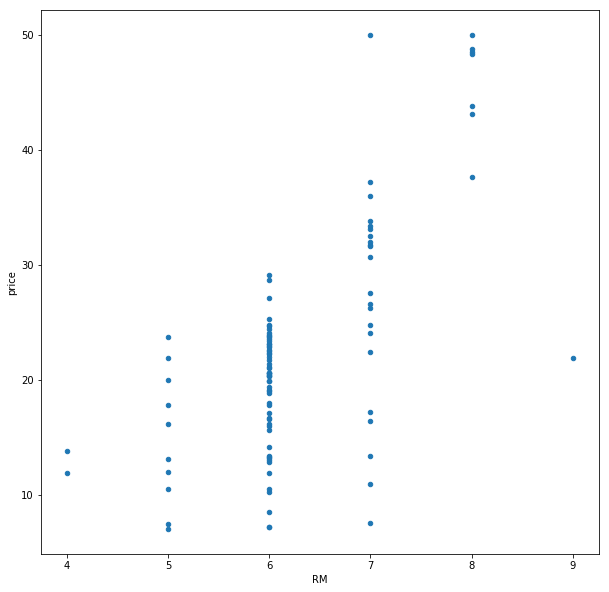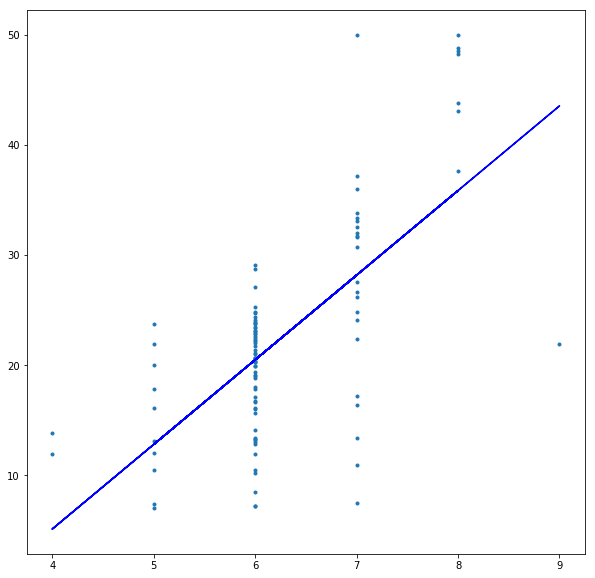# Precision of OLS Estimates

The calculation of the estimators and is based on sample data. As the sample drawn changes, the value of these estimators also changes. This leaves us with the question of how reliable these estimates are i.e. we’d like to determine the precision of these estimators. This can be determined by calculating the standard error or the coefficient of determination.

## Standard Error

The standard error of a statistic is the standard deviation of the sampling distribution of that statistic.

Suppose we have a dataset which contains incomes of people. From this dataset we start drawing samples of size n and calculating the mean. Now if we plot the distribution (the sampling distribution) of the means we calculated, we’ll get a normal distribution centered on the population mean. The standard deviation of this sampling distribution is the standard error of the statistic which in our case is mean.

Standard error is given by the formula: $\sigma_{\bar{x}} = \frac{\sigma}{\sqrt{n}}$

Where
is the standard deviation of the population.
is the sample size.

Calculating the standard error shows the sampling fluctuation. Sampling fluctuation shows the extent to which a statistic takes on different values.

Notice that the standard error has an inverse relation with the sample size n. This means that the larger the sample we draw from the population, the lesser the standard error will be. This will also result in a tighter normal distribution since the standard deviation will be less.

## Standard Error of OLS Estimates

The standard error of the OLS estimators and is given by:

where is the square root of true but unknown constant of homoscedastic variance .

All of the terms in the equations above except can be calculated from the sample drawn. Therefore, we will need an unbiased estimator . The denominator represents the degrees of freedom. is the residual sum of squares.

Although , its summation can be computed with an alternative formula as $\sum{\hat{u}_i^2} = \sum{y_i^2} - \frac{(\sum{x_iy_i})^2}{\sum{x_i^2}}$.

All of the terms in the above formula would have already been computed as a part of computing the estimators.

## How it all ties together

As you calculate the estimators and draw the sample regression curve that passes through your data, you need some numeric measure of how good the curve fits the data i.e. a measure of “goodness of fit”. This can be given as:

This is the positive square root of the estimator of homoscedastic variance. This is the standard deviation of the values about the regerssion curve.

The standard errors of the estimators and will show you how much they fluctuate as you draw the samples. The lesser their standard error, the better.

The square of the standard error is called the mean squared error.

## Let’s see some code

To start off, let’s load the Boston housing dataset.

I’m choosing to use the average number of rooms as the variable to see how it affects price. I’ve rounded it off to make the calculations easier. The scatter plot of the data looks the following:This, ofcourse, is the entire population data so let’s draw a sample.

Now let’s bring back our function that calculates the OLS estimates:

and now let’s see coefficients

The plot of the regression curve on the sample data looks the following:The sample DataFrame has our intermediate calculation and we can use that to calculate the standard error. Let’s write a function which does that.

Finally, let’s see how much standard deviation we have around the regression line.

## Coefficient of Determination

The coefficient of determination denoted by (for bivariate regression) or (for multivariate regression) is a ratio of the explained variance to the total variance of the data. The higher the coefficient of determination, the more accurately the regressors (average number of rooms in the house) explain the regressand (the price of the house) i.e. the better your regression model is.

For bivariate linear regression, value is given by:

can take on a value between 0 and 1.

Turning this into code, we have:

For the sample we’ve drawn, the value comes out to be 0.327. This means that only 32.7% of the variance in the total data is explained by the regression model.

Finito.

 A “statistic” is a numerical quantity such as mean, or median.